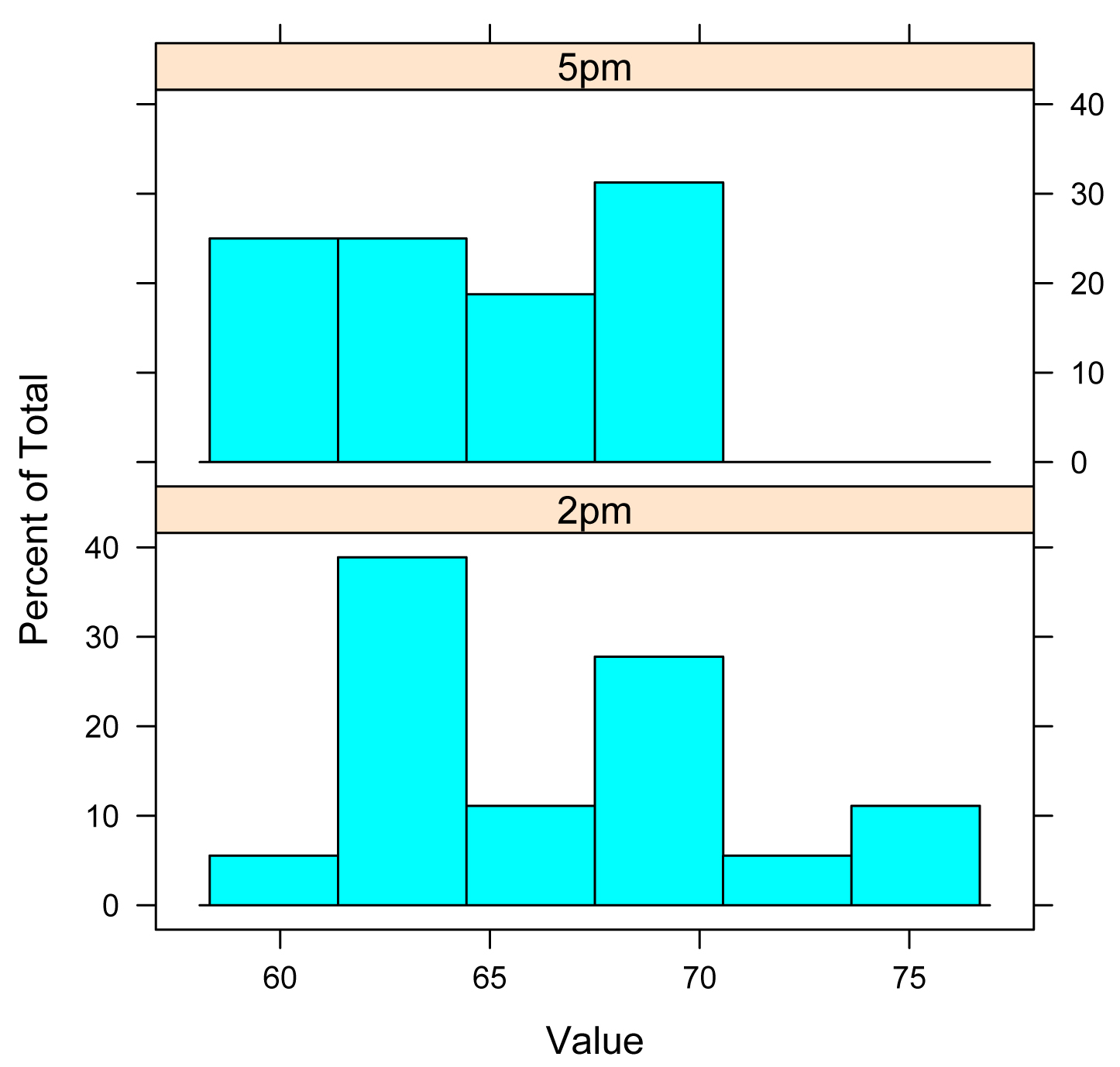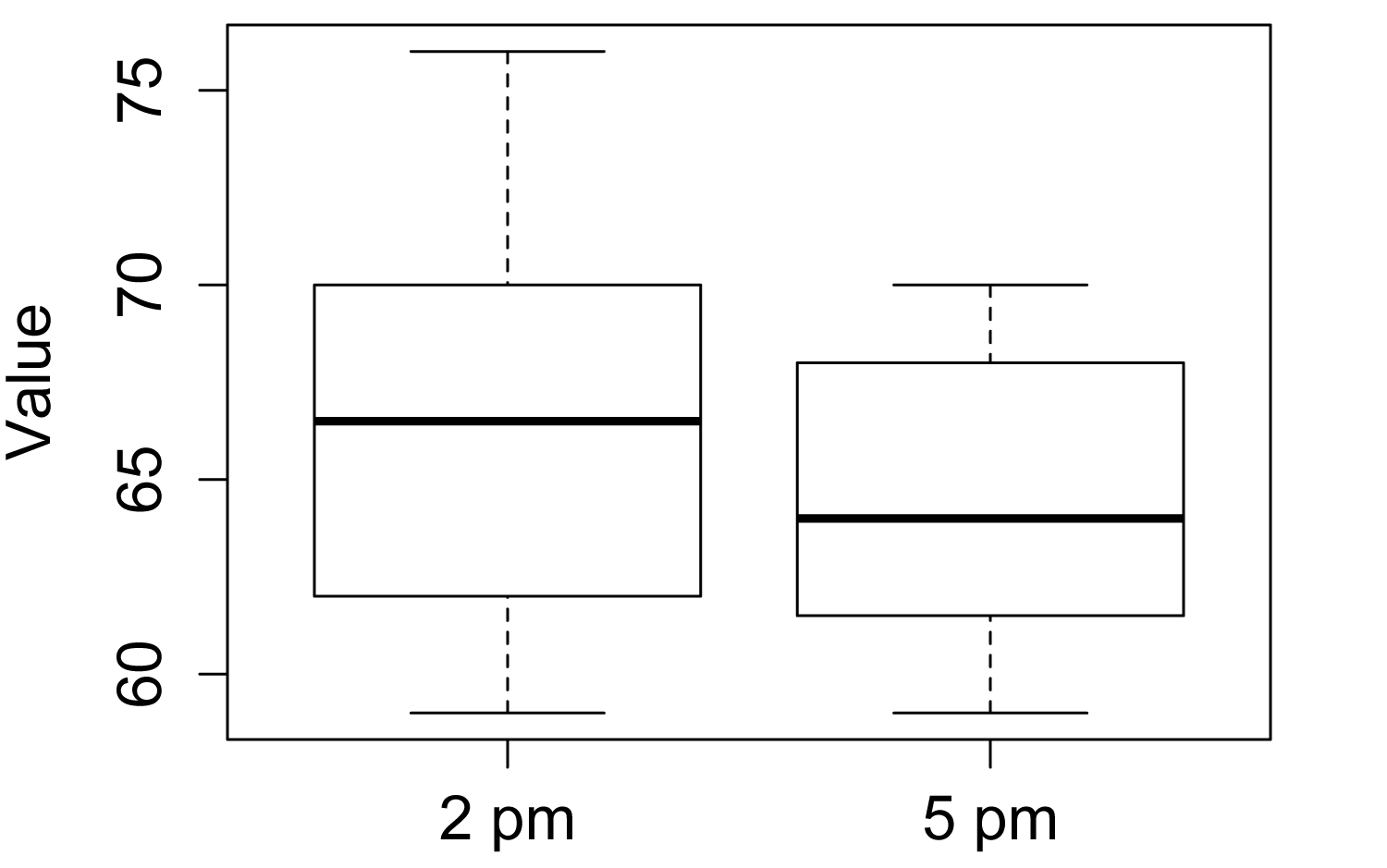## An R Companion for the Handbook of Biological Statistics

Salvatore S. Mangiafico

# Student’s t–test for Two Samples

Introduction

When to use it

Null hypothesis

How the test works

Assumptions

See the Handbook for information on these topics.

### Example

#### Two-sample t-test, independent (unpaired) observations

### --------------------------------------------------------------
### Two-sample t-test, biological data analysis class, pp. 128–129
### --------------------------------------------------------------

Input =("
Group Value
2pm    69
2pm    70
2pm    66
2pm    63
2pm    68
2pm    70
2pm    69
2pm    67
2pm    62
2pm    63
2pm    76
2pm    59
2pm    62
2pm    62
2pm    75
2pm    62
2pm    72
2pm    63
5pm    68
5pm    62
5pm    67
5pm    68
5pm    69
5pm    67
5pm    61
5pm    59
5pm    62
5pm    61
5pm    69
5pm    66
5pm    62
5pm    62
5pm    61
5pm    70
")

bartlett.test(Value ~ Group, data=Data)

### If p-value >= 0.05, use var.equal=TRUE below

Bartlett's K-squared = 1.2465, df = 1, p-value = 0.2642

t.test(Value ~ Group, data=Data,
var.equal=TRUE,
conf.level=0.95)

Two Sample t-test

t = 1.2888, df = 32, p-value = 0.2067

t.test(Value ~ Group, data=Data,
var.equal=FALSE,
conf.level=0.95)

Welch Two Sample t-test

t = 1.3109, df = 31.175, p-value = 0.1995

#### Plot of histograms

library(lattice)

histogram(~ Value | Group,
data=Data,
layout=c(1,2)      #  columns and rows of individual plots
)Histograms for each population in a two-sample t-test.  For the t-test to be valid, the data in each population should be approximately normal.  If the distributions are different, minimally Welch’s t-test should be used.  If the data are not normal or the distributions are different, a non-parametric test like Mann-Whitney U-test or permutation test may be appropriate.

#### Box plots

boxplot(Value ~ Group,
data = Data,
names=c("2 pm","5 pm"),
ylab="Value")Box plots of two populations from a two-sample t-test.

#     #     #

### Similar tests

Welch’s t-test is discussed below.  The paired t-test and signed-rank test are discussed in this book in their own chapters. Analysis of variance (anova) is discussed in several subsequent chapters.

As non-parametric alternatives, the Mann–Whitney U-test and the permutation test for two independent samples are discussed in the chapter Mann–Whitney and Two-sample Permutation Test.

#### Welch’s t-test

Welch’s t-test is shown above in the “Example” section (“Two sample unpaired t-test”).  It is invoked with the var.equal=FALSE option in the t.test function.

How to do the test

The SAS example from the Handbook is shown above in the “Example” section.

### Power analysis

#### Power analysis for t-test

### --------------------------------------------------------------
### Power analysis, t-test, wide feet, p. 131
### --------------------------------------------------------------

M1  = 100.6                      # Mean for sample 1
M2  = 103.6                      # Mean for sample 2
S1  =  5.26                      # Std dev for sample 1
S2  =  5.26                      # Std dev for sample 2

Cohen.d = (M1 - M2)/sqrt(((S1^2) + (S2^2))/2)

library(pwr)

pwr.t.test(
n = NULL,                   # Observations in _each_ group
d = Cohen.d,
sig.level = 0.05,           # Type I probability
power = 0.90,               # 1 minus Type II probability
type = "two.sample",        # Change for one- or two-sample
alternative = "two.sided")

Two-sample t test power calculation

n = 65.57875     # Number for each group

#     #     #